# Implicit differentiation Implicit differentiation is used to differentiate

• Slides: 26Implicit differentiation.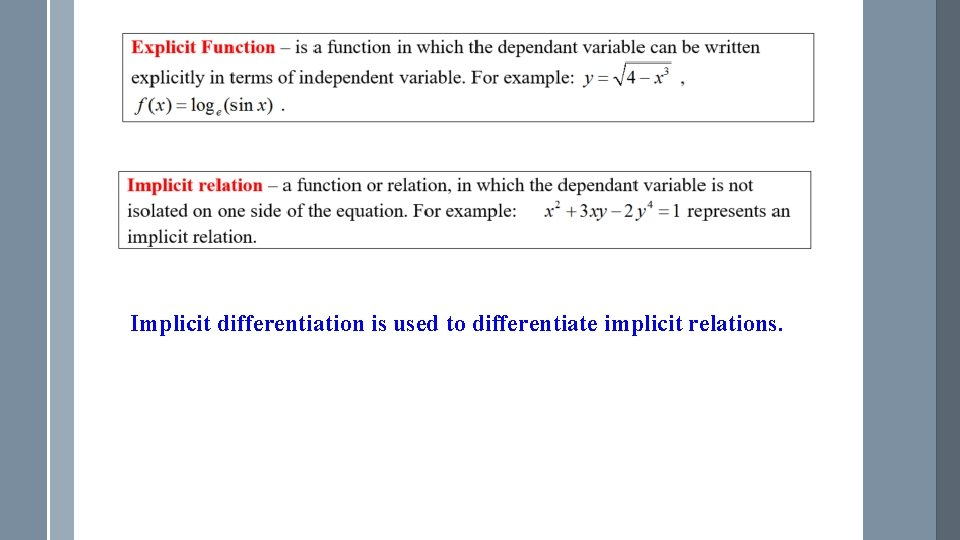Implicit differentiation is used to differentiate implicit relations.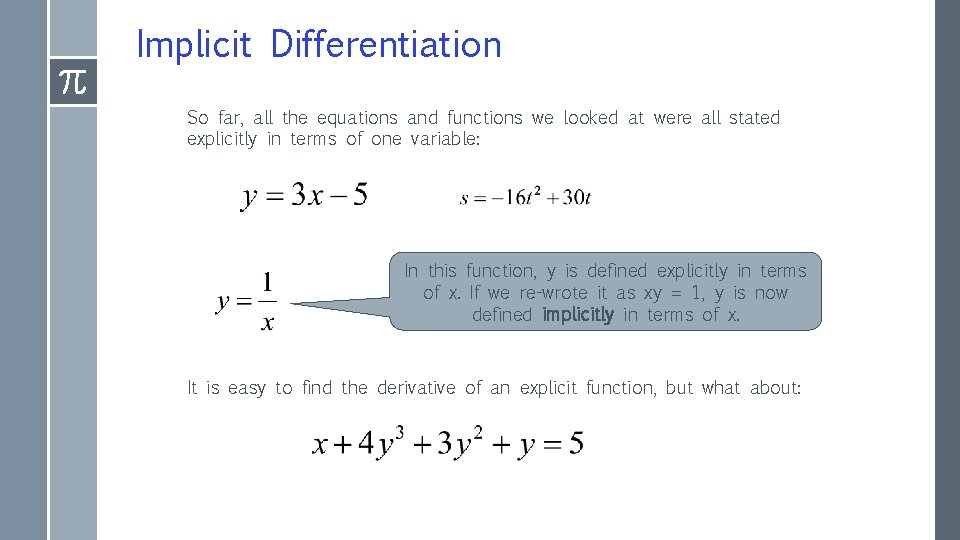Implicit Differentiation So far, all the equations and functions we looked at were all stated explicitly in terms of one variable: In this function, y is defined explicitly in terms of x. If we re-wrote it as xy = 1, y is now defined implicitly in terms of x. It is easy to find the derivative of an explicit function, but what about: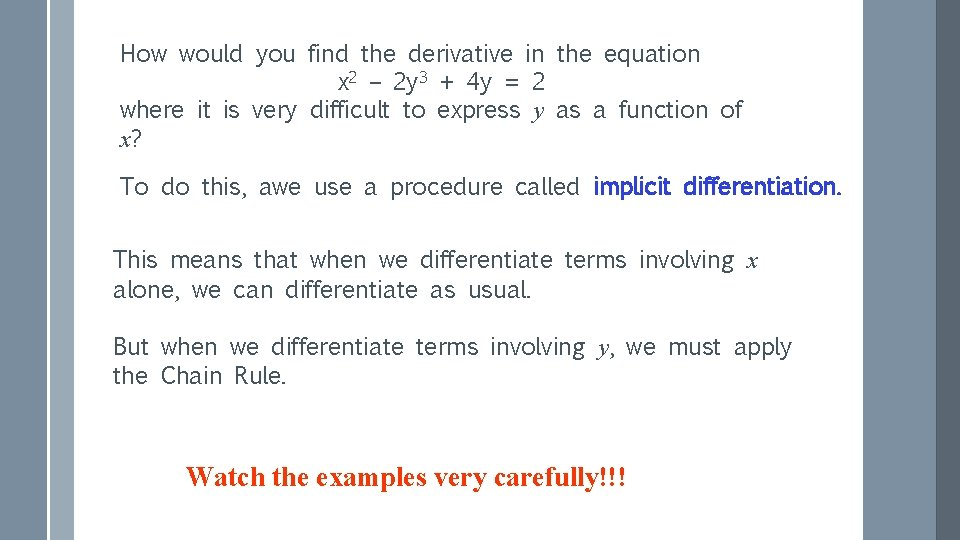How would you find the derivative in the equation x 2 – 2 y 3 + 4 y = 2 where it is very difficult to express y as a function of x? To do this, awe use a procedure called implicit differentiation. This means that when we differentiate terms involving x alone, we can differentiate as usual. But when we differentiate terms involving y, we must apply the Chain Rule. Watch the examples very carefully!!!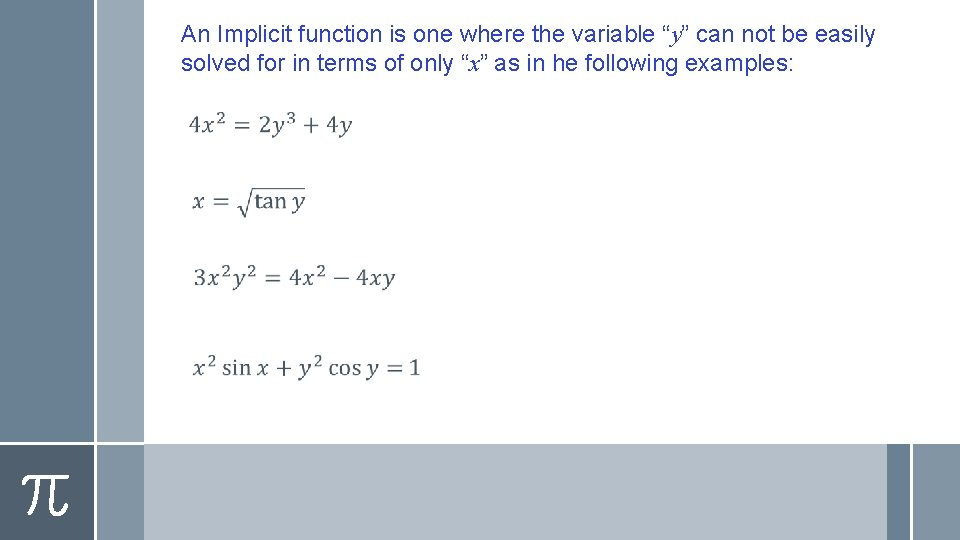An Implicit function is one where the variable “y” can not be easily solved for in terms of only “x” as in he following examples: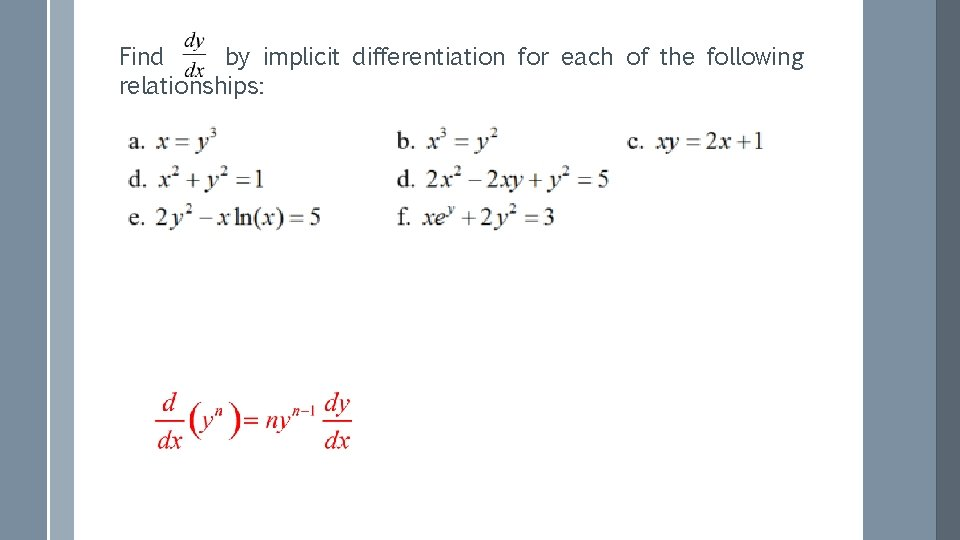Find by implicit differentiation for each of the following relationships:We differentiate each term Whenever we differentiate a term with y we need to have dy/dx next to it.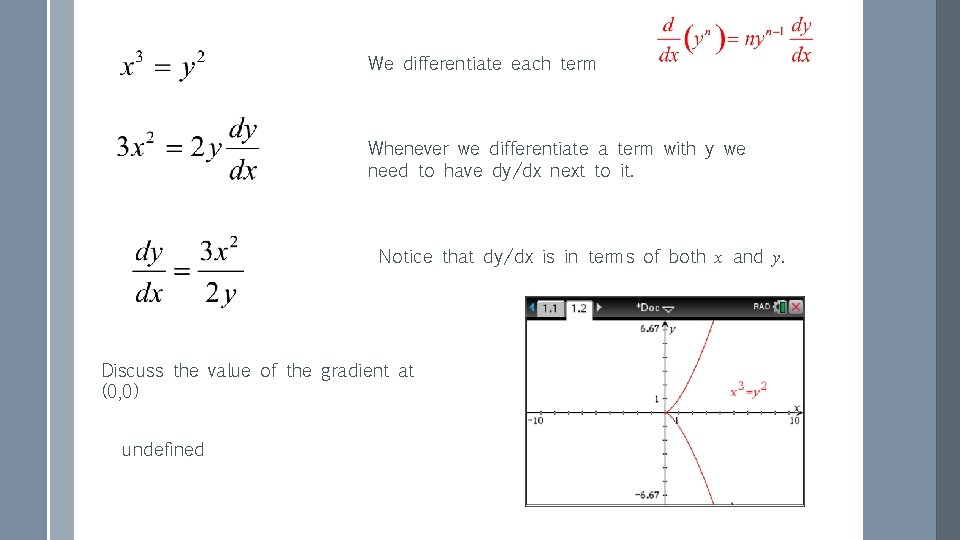We differentiate each term Whenever we differentiate a term with y we need to have dy/dx next to it. Notice that dy/dx is in terms of both x and y. Discuss the value of the gradient at (0, 0) undefined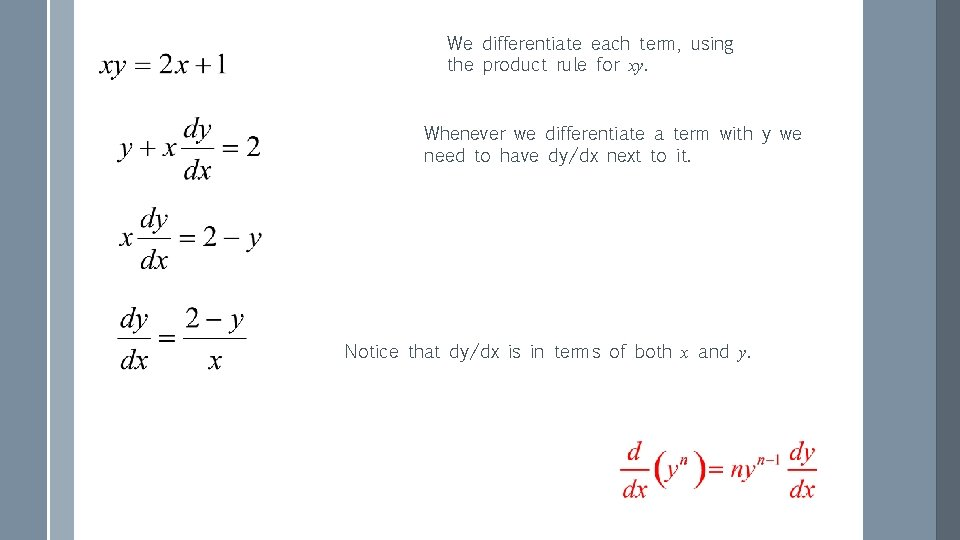We differentiate each term, using the product rule for xy. Whenever we differentiate a term with y we need to have dy/dx next to it. Notice that dy/dx is in terms of both x and y.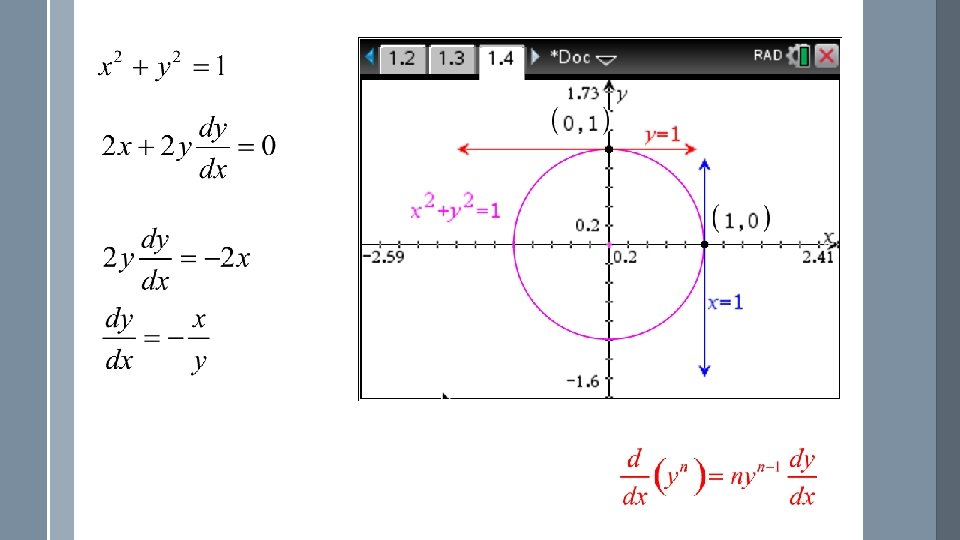We differentiate each term Whenever we differentiate a term with y we need to have dy/dx next to it. It is a circle. Discuss vertical and horizontal tangents.We differentiate each term, using the product rule for 2 xy. Whenever we differentiate a term with y we need to have dy/dx next to it. Vertical tangents:(a) by explicit differentiation (making y the subject). (b) by implicit differentiation.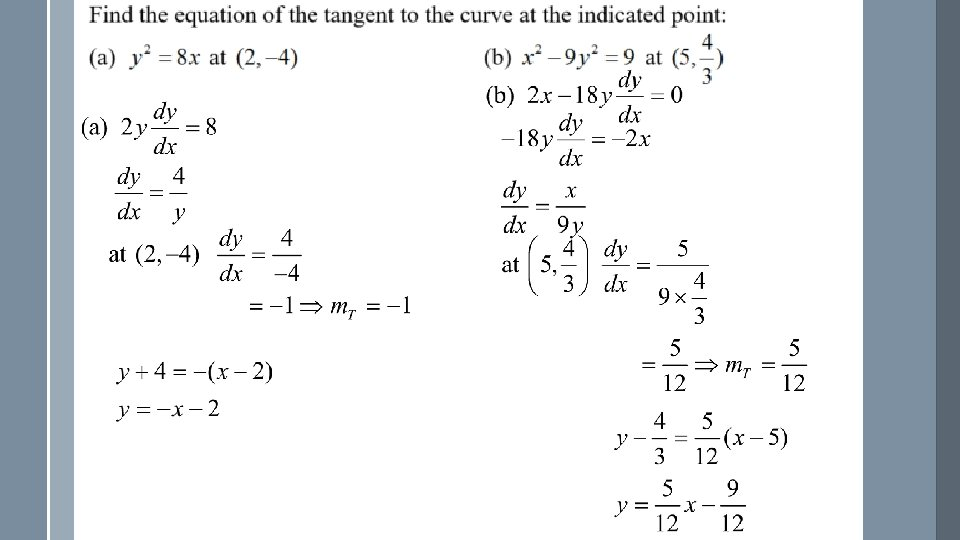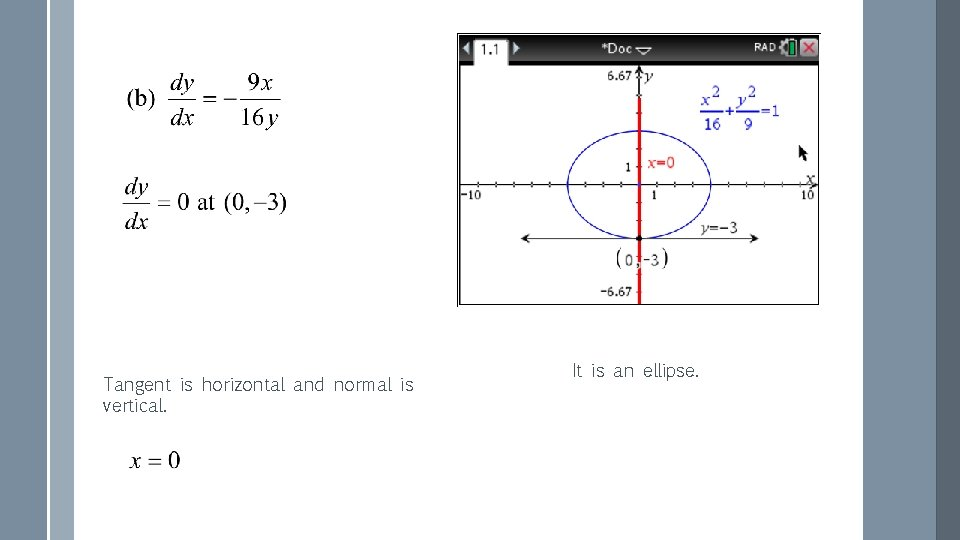Tangent is horizontal and normal is vertical. It is an ellipse.Find dy/dx given that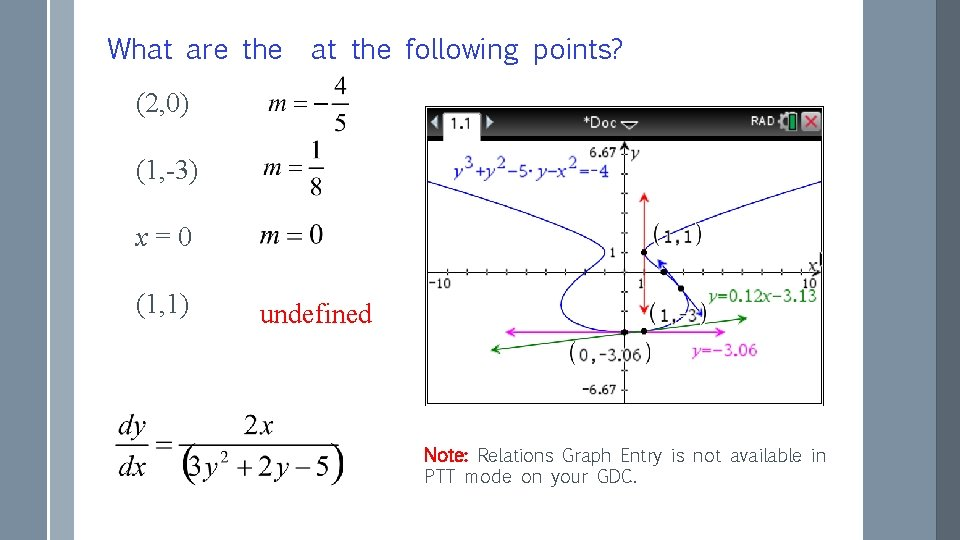What are the at the following points? (2, 0) (1, -3) x=0 (1, 1) undefined Note: Relations Graph Entry is not available in PTT mode on your GDC.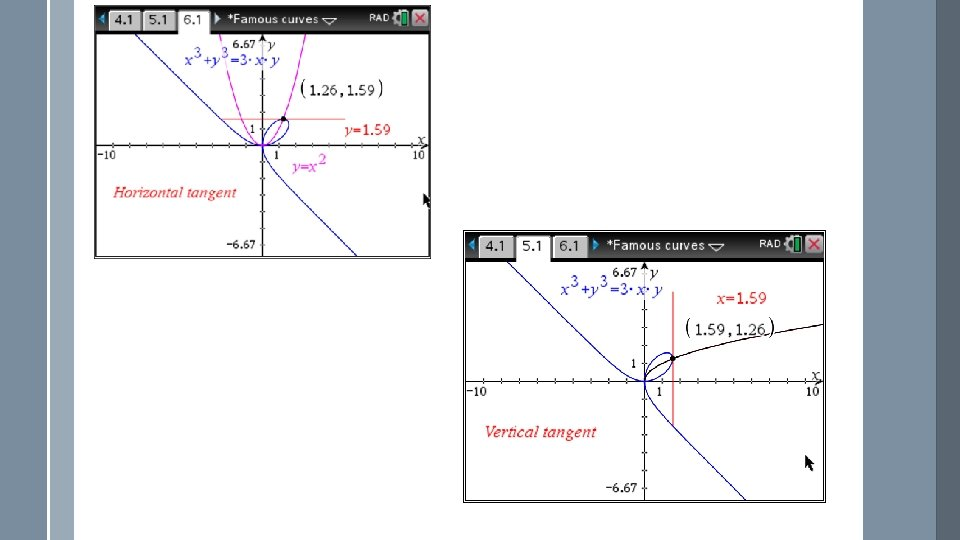Find the equation of the tangent to the Folium of Descartes at (2, 4).This can’t be solved for y.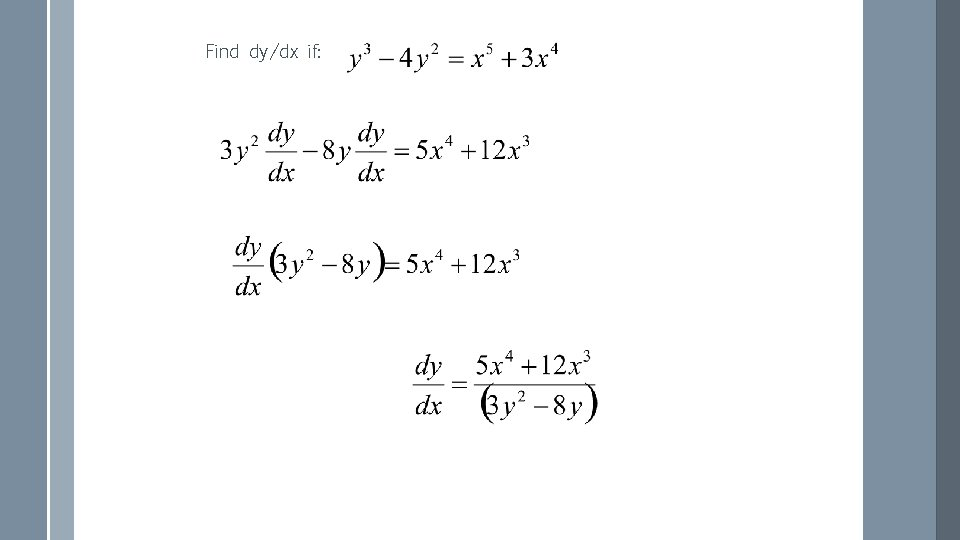Find dy/dx if:Find dy/dx if: Chain Rule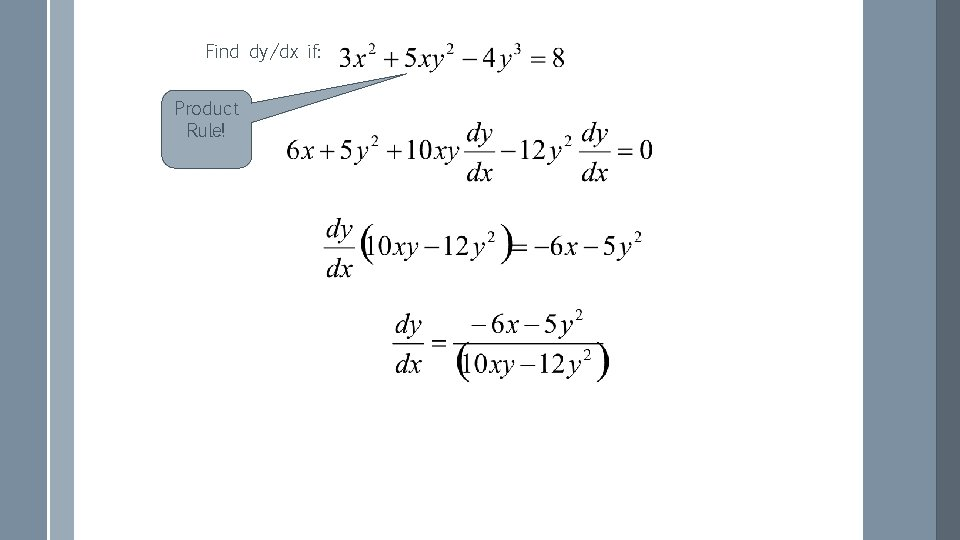Find dy/dx if: Product Rule!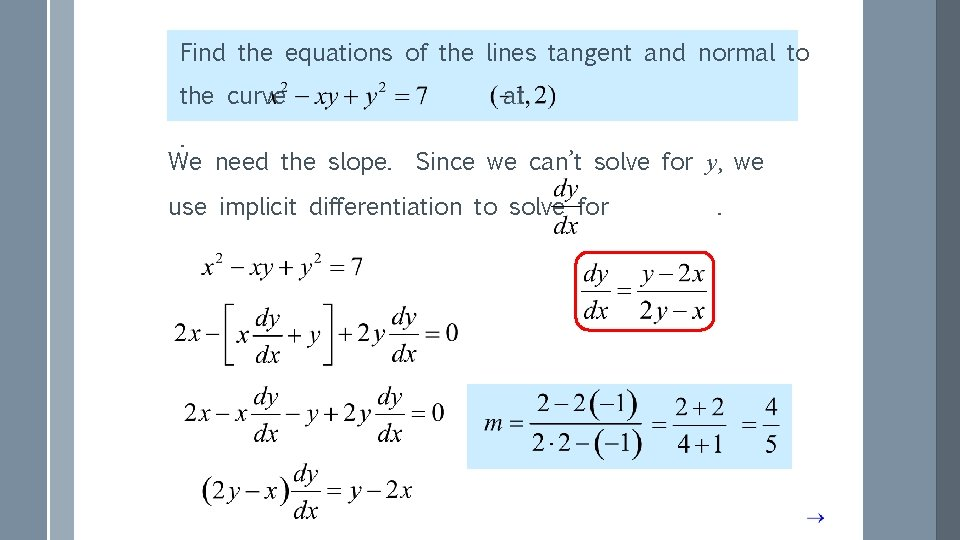Find the equations of the lines tangent and normal to the curve. We need the slope. at Since we can’t solve for y, we use implicit differentiation to solve for Note product rule. .Find the equations of the lines tangent and normal to the curve at . tangent: normal: Normal line is perpendicular to tangent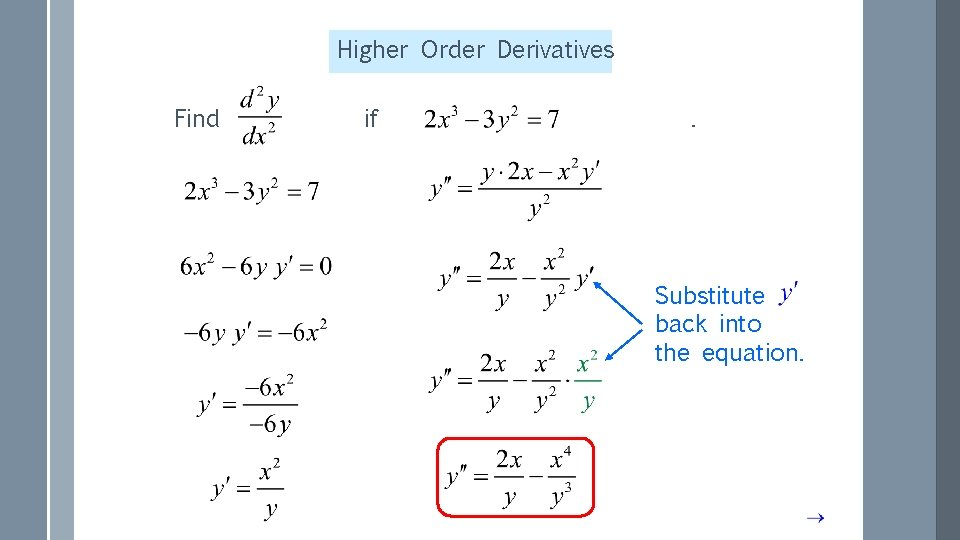Higher Order Derivatives Find if . Substitute back into the equation.